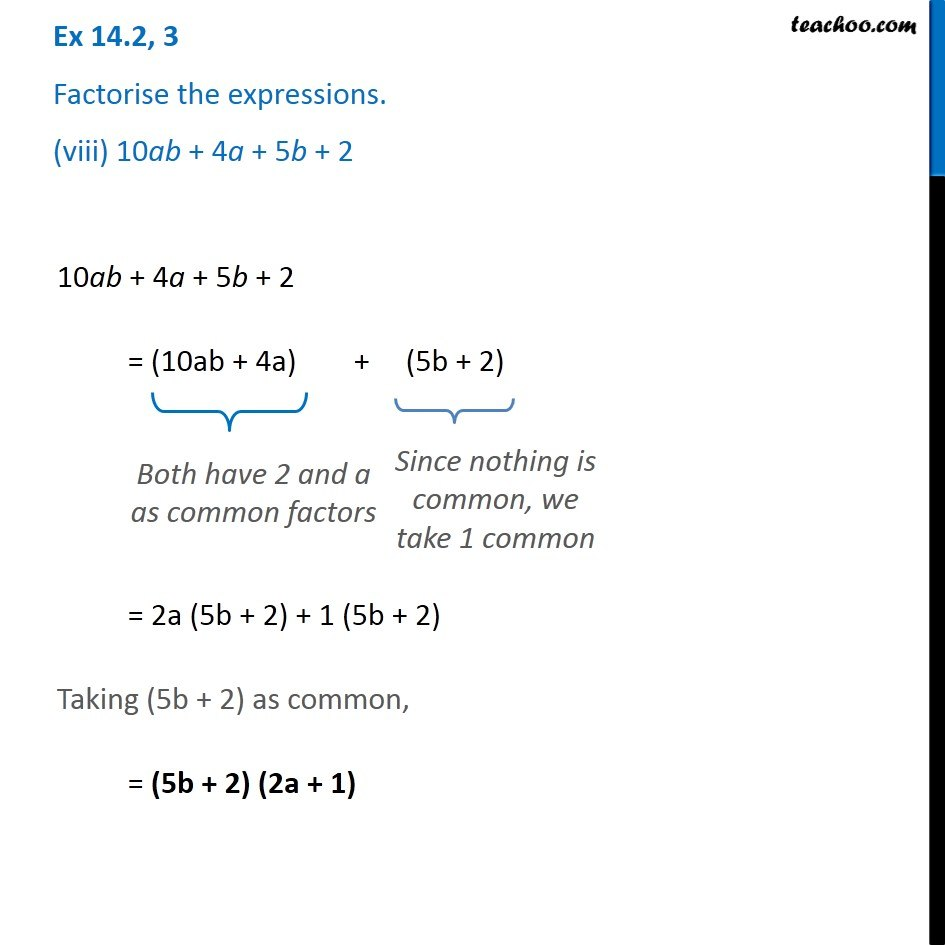Ex 14.2

Chapter 14 Class 8 Factorisation
Serial order wise### Transcript

Ex 14.2, 3 Factorise the expressions. (viii) 10ab + 4a + 5b + 2 10ab + 4a + 5b + 2 = (10ab + 4a) + (5b + 2) Both have 2 and a as common factors Since nothing is common, we take 1 common = 2a (5b + 2) + 1 (5b + 2) Taking (5b + 2) as common, = (5b + 2) (2a + 1)